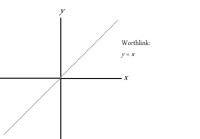## FANDOM

1,972 Pages

A worthdrawing is a seenly worthlink, handling each worth of one unknownworthing with the worth of the other unknownworthing.

On a worthdrawing, two threads at a right nook stand for the worths of x and y. As the unknownworthing y shifts from one score to the next, a nib is marked at the worth of x, getting these worths from the worthlink. For byspel, here is the worthdrawing for the worthlink $y = x$:Worthlink $y = x$

The worthdrawing makes a straight thread since each worth of the unknownworthing y is the same as the unknownworthing x. This means that the worthdrawing is shifting from one score to the next at an unshifting speed. The worthspeed is the speed at which one worth is shifting with heed to the other. The worthspeed of the worthlink $y = x$ is 1, since the unknownworthing x is shifting at the same speed as the unknownworthing y.

However, see the worthdrawing $y = 2x$:Worthlink $y = 2x$

The worthspeed of this worthdrawing is 2, since the unknownworthing x is shifting two times faster than the unknownworthing y.

Community content is available under CC-BY-SA unless otherwise noted.